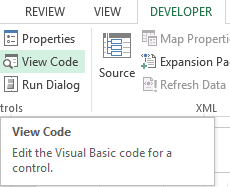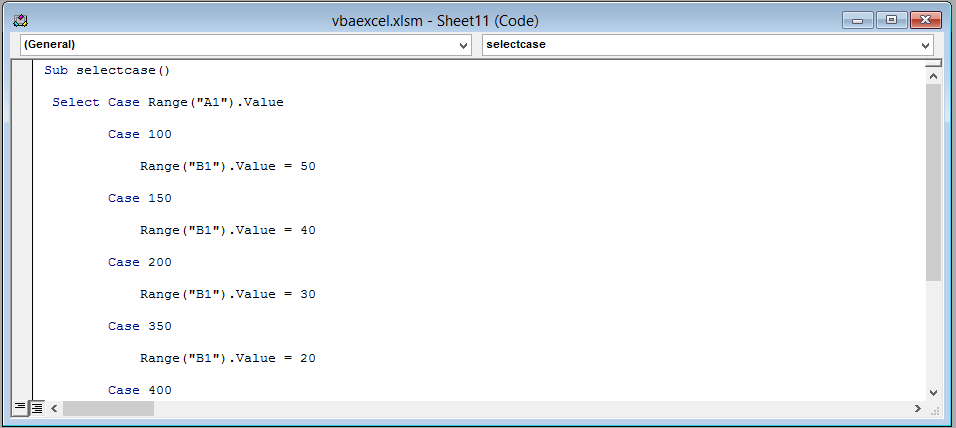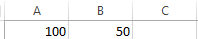### Select Case in Excel VBA

In this article you are going to learn how to use the select case statement. The SELECT CASE statement just like the IF STATMENT is a condition.

Conditions are very useful in programming because they allow the user to execute actions based on specific criteria (that the user defines).

The SELECT CASE STATEMENT just like the IF STATEMENT is used when the user wants to check one or more than one conditions. This is another method to make a decision based on a specific criteria.

SELECT CASE STATEMENT is very flexible and easy to read and use (some people prefer using the SELECT CASE statement instead of the IF statement. The SELECT CASE statement is an alternative to using IF statement with lots of Else If instructions: the Select command, which is better suited to these sorts of situations.

```Select Case
Case
Do something
Case
Do something
Case
Do something
Case Else
Do something else
End Select
```

Note: A SELECT CASE statement just like the IF STATEMENT can have as many cases as you like. Case<Test1>, Case<Test2> is the same as of writing Else If in the IF STATEMENT.

Explanation: First you write SELECT CASE followed by a expression you would like to test. After that you write Case followed by a test like Case 1 or Case A. After this you define what will happen in Case A. To end the SELECT CASE statement you write End Select.

Click on Developer tab and select “View CodeA new window (Visual Basic Editor) will open which would have a dialog box in the center. You will write the code in the dialog box.Write the following line of code in the dialog box.

```Sub selectcase ()
Select Case Range ("A1").Value
Case 100
Range ("B1").Value = 50
Case 150
Range ("B1").Value = 40
Case Else
Range ("B1").Value = 0
End Select
End Sub```

After writing the code close the window by clicking on the cross(x) icon on the upper right side of the screen.

Explanation: In this code we are simple changing the value in cell B if there is a certain value in cell A. For example if the user entered 100 in cell A1 than the value in cell B1 will be 50. Like this if the user changes the value in cell A1 to 150 than the value in cell B1 would be 40. “Case Else” means that if none of the above condition are true (meaning if the values in cell A1 isn’t 100,150) than the value in cell b1 would be 0. End Select is used to end the SELECT CASE statement.This is the result if the user has entered 100 in cell A1.This is the result if the user has entered 150 in cell A1.This is the result if the user has entered any value in cell A1 except 100 or 150.That’s it you have now learned how to use a SELECT CASE statement in Excel VBA

## Template

```Further reading: